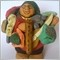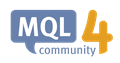# How to confirm breakout trades with volume in code?96

Hi,

Many professional traders recommend using volume to confirm breakouts. They say one can confirm a breakout when there is a spike in volume. I am looking to test this in an ea but can't translate it into code exactly. Now, how would one quantify a "spike" to put implement this in code? When price breaks out of a defined range, I tried taking indicator volume value at shift 1 and indicator volume value at shift 2, and if shift 1 value is higher than shift 2 then this means that volume has increased. This increase can be called a "spike" but this results in many fakeouts. Or is trading breakouts strictly a discretionary technique?2102

Dwaine Hinds:

Hi,

Many professional traders recommend using volume to confirm breakouts. They say one can confirm a breakout when there is a spike in volume. I am looking to test this in an ea but can't translate it into code exactly. Now, how would one quantify a "spike" to put implement this in code? When price breaks out of a defined range, I tried taking indicator volume value at shift 1 and indicator volume value at shift 2, and if shift 1 value is higher than shift 2 then this means that volume has increased. This increase can be called a "spike" but this results in many fakeouts. Or is trading breakouts strictly a discretionary technique?

I doubt MT5 gives real volume values. Perhaps volume data the broker or a selected liquidity provider. Confirm this with your broker.

Like MT4, I think MT5 just shows the number of ticks arrived (number of price updates). I'm not sure if this data can be used to confirm breakouts. I read somewhere of people using volume data from forex futures obtained from a third party vendor96

I am ware that I will not get real volume data in forex because it's so decentralized. I am ok with tick volume because its better than nothing. I have reconstructed an indicator to give volume weighted moving average and its working alright.

Volume_Weighted_MA.mq4 :

```//+------------------------------------------------------------------+
//|                                           Volume_Weighted_MA.mq4 |
//|                                                      MT4 TO |
//|                                                                  |
//+------------------------------------------------------------------+
#property copyright "Community TO"
#property link      "http://www.mql4.com"

#property indicator_separate_window//indicator_chart_window
#property indicator_buffers 1
#property indicator_color1 DeepPink

extern string               aSymbol = "";
extern ENUM_TIMEFRAMES      ChartTimeframe=0;
extern int                  Period_MA=21;
extern ENUM_APPLIED_PRICE   Price_MA = PRICE_MEDIAN; //Price type
//extern int  Price_MA=PRICE_MEDIAN;
extern int                  Shift=1;

double MABuffer[];
double Vol;
//+------------------------------------------------------------------+
//|                                                                  |
//+------------------------------------------------------------------+
int init()
{
IndicatorBuffers(1);
SetIndexShift(0,0);
SetIndexDrawBegin(0,Period_MA);
SetIndexBuffer(0,MABuffer);
SetIndexStyle(0,DRAW_HISTOGRAM);//SetIndexStyle(0,DRAW_LINE);
IndicatorDigits(Digits+1);

ArrayResize(Vol,Period_MA);

return(0);
}
//+------------------------------------------------------------------+
//|                                                                  |
//+------------------------------------------------------------------+
int start()
{
int limit;
int j,i;
double sum;
double Price;

int counted_bars=IndicatorCounted();
if(counted_bars<0) return(-1);
if(counted_bars>0) counted_bars--;
limit=iBars(aSymbol,ChartTimeframe)-counted_bars;
if(counted_bars==0) limit-=Period_MA;

for(i=Shift; i<limit; i++)//for(i=0; i<limit; i++)
{
sum=0;
Price=0;
for(j=i;j<i+Period_MA;j++)
{
Vol[j-i]=iVolume(aSymbol,ChartTimeframe,j);//Volume[j];
sum+=Vol[j-i];
}
for(j=0;j<Period_MA;j++)
{
Vol[j]/=sum;
}
for(j=i;j<i+Period_MA;j++)
{
Price+=get_price(j,Price_MA,aSymbol,ChartTimeframe)*Vol[j-i];
}
MABuffer[i]=Price;
}

return(0);
}
//+------------------------------------------------------------------+
//|                                                                  |
//+------------------------------------------------------------------+
double get_price(int num,int applied_price,string asymbol,ENUM_TIMEFRAMES acharttimeframe)
{
switch(applied_price)
{
case  PRICE_CLOSE    :  return (iClose(asymbol,acharttimeframe,num));//return(Close[num]);
case  PRICE_OPEN     :  return (iOpen(asymbol,acharttimeframe,num));//return(Open[num]);
case  PRICE_HIGH     :  return (iHigh(asymbol,acharttimeframe,num));//return(High[num]);
case  PRICE_LOW      :  return (iLow(asymbol,acharttimeframe,num));//return(Low[num]);
case  PRICE_MEDIAN   :  return ( (iHigh(asymbol,acharttimeframe,num) + iLow(asymbol,acharttimeframe,num))/2 );//return((High[num]+Low[num])/2);
case  PRICE_TYPICAL  :  return ( (iHigh(asymbol,acharttimeframe,num) + iLow(asymbol,acharttimeframe,num) + iClose(asymbol,acharttimeframe,num))/3 );//return((High[num]+Low[num]+Close[num])/3);
case  PRICE_WEIGHTED :  return ( (iHigh(asymbol,acharttimeframe,num) + iLow(asymbol,acharttimeframe,num) + iClose(asymbol,acharttimeframe,num) + iClose(asymbol,acharttimeframe,num))/4 );//return((High[num]+Low[num]+Close[num]+Close[num])/4);
default              :  return ( (iHigh(asymbol,acharttimeframe,num) + iLow(asymbol,acharttimeframe,num))/2 );//return((High[num]+Low[num])/2);
}
}
//+------------------------------------------------------------------+
```

VWMA_Indicator.mqh:

```//+------------------------------------------------------------------+
//|                                               VWMA_Indicator.mqh |
//|                        Copyright 2019, Everybodys' Software Corp. |
//|                                       https://www.somewhere.com |
//+------------------------------------------------------------------+
#property copyright "Copyright 2019, Everybodys' Software Corp."
#property link      "https://www.somewhere.com"
#property strict

//+------------------------------------------------------------------+
// iVWMA
//+------------------------------------------------------------------+
/*
This gets the current volume weighted moving average value.
*/
double iVWMA(string vSymbol, ENUM_TIMEFRAMES vChartTimeframe, int vPeriod, ENUM_APPLIED_PRICE vAppliedPrice, int vShift)
{
double vwma=0;
vwma = iCustom(vSymbol,vChartTimeframe,"Volume_Weighted_MA",vSymbol,vChartTimeframe,vPeriod,vAppliedPrice,vShift,0,vShift);
return vwma;
}
//+------------------------------------------------------------------+
// End of iVWMA
//+------------------------------------------------------------------+

```

Example usage of 20 period:

`double aVWMA1 = iVWMA(NULL,0,20,PRICE_MEDIAN,1);//shift 1 volume weighted moving average`

This indicator does not have a problem I am aware of. Hoping someone could provide alternative ways of confirming breakouts.MQL4: automated forex trading, strategy tester and custom indicators with MetaTrader
• www.mql4.com
MQL4: automated forex trading, strategy tester and custom indicators with MetaTrader
To add comments, please log in or register